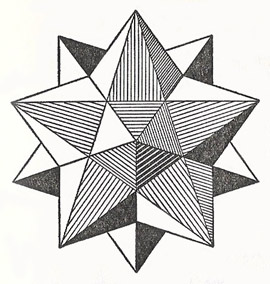A# dodecahedronFigure 1. Net of a dodecahedron.

A dodecahedron is a polyhedron with 12 faces. A regular dodecahedron is made from faces that are identical regular pentagons and is one of the Platonic solids.

In the case of rhombic dodecahedra, the twelve faces are twelve rhombuses, and in the case of pentagon dodecahedra (pentadodecahedra) twelve regular pentagons.Figure 2. A cube inside a dodecahedron.

If the edges of pentadodecahedron are extended, a new solid arises called the dodecahedron-star (Fig 3).Figure 3. Dodecadron-star.